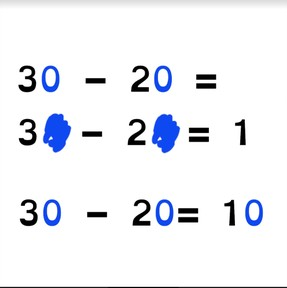Subtraction to 100 via the zero rule

# Subtraction to 100 via the zero rule8,000 schools use Gynzy92,000 teachers use Gynzy1,600,000 students use Gynzy

## General

Students learn to subtract tens to 100 using the zero-rule. They learn to subtract tens quickly by making a first step of subtracting the numbers without the zeros.

2.NBT.B.5

## Relevance

When students learn to subtract numbers to 100 using the zero rule, they can subtract larger numbers quicker.

## Introduction

Divide the class into two groups and have the two lines form parallel to each other. Name a subtraction problem using numbers less than 10 and ask the two students at the front for the difference. Only the two students at the front of the line may answer. The student who gives the correct answer first wins a point for their team. The first two students may now sit down, repeat this using different subtraction problems until all students have had a turn. The team with the most points wins eternal fame.

## Development

Discuss that it is important to learn how to work with the zero rule because it helps you subtract easier and quicker. Remind students that tens are a group of ten and that they end in a 0. Ask students if they can name tens numbers. Then show the zero rule with the subtraction problems of 2-1 and 20+10 with the box of chocolates as well as MAB blocks to serve as visual support. Next show the subtraction problem of 30 - 20 = 10 and ask students to drag the covers over the zeros. Explain that this shows their first step of subtraction. Then ask them to erase the zeros on the next subtraction problem. They uncover the subtraction problem with zeros and can solve.
Next explain the zero rule with an example. You show that when you have a subtraction problem with tens that you can create a first step by taking away the zeros. When you do this, you make the problem ten times smaller. If you have a zero in both of the numbers, then the answer also gets a zero. Explain to students that if you already have the first step, that you can use this because the answer simply becomes ten times larger when you add a zero to the end. Practice the zero rule with students, making sure to ask what the first step is before solving the problem. Also be sure to mention that the problem becomes ten times smaller or ten times larger when taking away and adding the zero.

Check that students understand subtraction using the zero rule by asking the following questions:
- Which numbers do you use to create the first step?
- What do you do with tens numbers that you want to subtract quickly and easily?
- What happens with the answer if both numbers have zeros?

## Guided practice

Students practice questions in which the first step is given, and then they must determine what the first step is, and are given variation in smaller and larger numbers to subtract.

## Closing

Check that students are able to subtract tens from numbers to 100 using the zero rule by asking students to explain the steps to subtract two numbers using the zero rule. Then ask them to explain the strategy of subtracting a problem at random like 50 - 20. To close the lesson, play the game that you started the lesson with, but use subtraction problems to 100.

## Teaching tips

To challenge your students- during the closing game you may also ask your students to name the first step of subtraction rather than the difference.

### The online teaching platform for interactive whiteboards and displays in schools

• Save time building lessons

• Manage the classroom more efficiently

• Increase student engagement